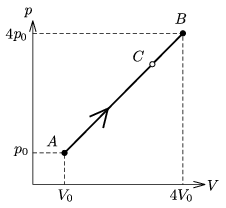Mathematical and Physical Journal
for High Schools
Issued by the MATFUND Foundation
 Already signed up? New to KöMaL?

#Problem P. 4049. (February 2008)

P. 4049. A sample of diatomic gas of certain mass is taken from state A to state B as shown in the figure; p0=105 Pa, V0=2 dm3.

a) Determine the pressure and the volume of the gas at the intermediate state C until which only QAC=3150 J heat was added to the gas.

b) By what factor does the internal energy of the gas changed during process AC?

c) How much work was done by the expanding gas during process AC?(4 pont)

Deadline expired on March 11, 2008.

### Statistics:

 120 students sent a solution. 4 points: 73 students. 3 points: 8 students. 2 points: 25 students. 1 point: 11 students. 0 point: 3 students.

Problems in Physics of KöMaL, February 2008